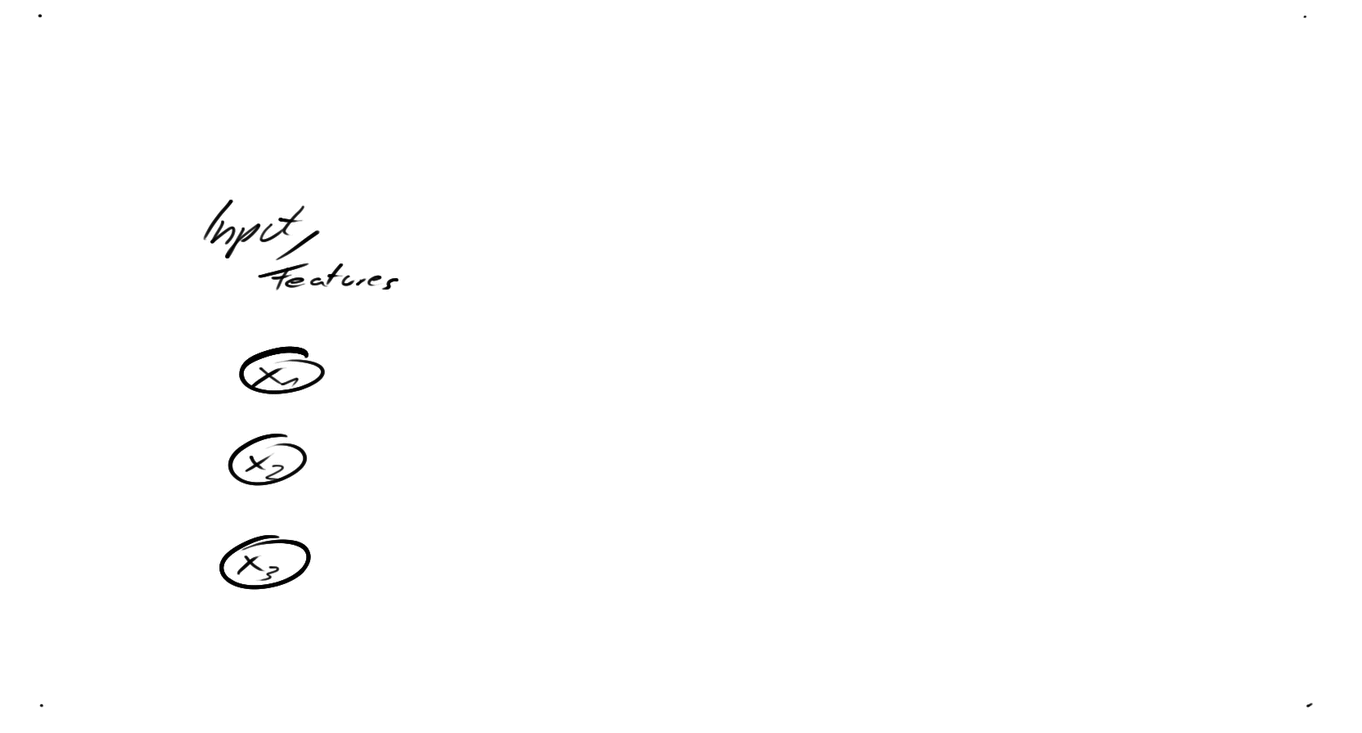# Introduction to Neural Networks

In Introduction to Machine Learning you have learned about the linear and logistic regregression to predict continous values or classes. You have learned about activation functions (sigmoid function), cost functions (mean squared error, cross-entropy) and how to iteratively reduce the costs by optimizing your model parameters with gradient descent.

In this course you will extend the logistic regression model to a fully connected neural network in order to be able solve non-linear serperable classification tasks. To accomplish this, you will learn about the backpropagation algorithm and implement it.## Exercises

In Introduction to Machine Learning(highly recommended), you calulated the gradient by hand and just used the final formula. In this exercise you will learn how to just derive the single individual functions and chain them programatically. This allows to programmatically build computational graphs and derive them w.r.t. certain variables, only knowing the derivatives of the most basic functions.

Here you will learn to visualize a neural network given matrices of wheights and compute the forward pass using matrix and vector operations.

Knowing how to compute the forward pass and the backward pass with backpropagation, you are ready for a simple neural neutwork. First you will refresh your knowledge about logistic regression, but this time, implement it using computational graph. Then you will add a hidden layer. Further you will understand what happens with the data in the hidden layer by plotting it.

For a better understanding of neural networks, you will start to implement a framework on your own. The given notebook explains some core functions and concepts of the framework, so all of you have the same starting point. Our previous exercises were self-contained and not very modular. You are going to change that. Let us begin with a fully connected network on the now well-known MNIST dataset. The Pipeline will be

After this course you can go on either with the CNN course or the Differential-Programming course.

## Reference (ISO 690)

 [GLO10] GLOROT, Xavier; BENGIO, Yoshua. Understanding the difficulty of training deep feedforward neural networks. In: Proceedings of the thirteenth international conference on artificial intelligence and statistics. 2010. S. 249-256.